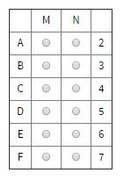## A fair coin has 2 distinct flat sides-one of which bears t

##### This topic has expert replies
Legendary Member
Posts: 1723
Joined: 29 Oct 2017
Followed by:6 members

### A fair coin has 2 distinct flat sides-one of which bears t

by swerve » Wed May 01, 2019 1:43 pm
A fair coin has 2 distinct flat sides-one of which bears the image of a face and the other of which does not-and when the coin is tossed, the probability that the coin will land faceup is $$\frac{1}{2}$$. For certain values of M, N, p, and q, when M fair coins are tossed simultaneously, the probability is p that all M coins land faceup, and when N fair coins are tossed simultaneously, the probability is q that all N coins land face up. Furthermore, $$M < N$$ and $$\frac{1}{p} + \frac{1}{q} = 72$$.

In the table, select a value for $$M$$ and a value for $$N$$ that are jointly consistent with the given information. Make only two selections, one in each column.The OA is [spoiler]M=3 N=6[/spoiler]

Source: Official Guide

### GMAT/MBA Expert

GMAT Instructor
Posts: 2094
Joined: 04 Dec 2012
Thanked: 1443 times
Followed by:245 members
by ceilidh.erickson » Sat Jun 01, 2019 9:58 am
If one coin flip has a 1/2 chance of landing face up, then the probability that two coin flips will both be face up is $$\frac{1}{2}\cdot\frac{1}{2}=\frac{1}{4}$$

Thus, for any M coin flips, the probability of all flips landing face up is $$p=\frac{1}{2^M}$$ , and for any N coin flips, the probability of all flips landing face up is $$q=\frac{1}{2^N}$$ .

We're told that $$\frac{1}{p} + \frac{1}{q} = 72$$ , so we can infer that $${2^M}+{2^N}=72$$ . Think of powers of 2 that would add to 72, starting with the largest power of 2 less than 72:
$${2^6}$$ --> 64 + 8 = 72 --> yes! These are both powers of 2.
$${2^5}$$ --> 32 + 40 = 72 --> no, 40 is not a power of 2.
$${2^4}$$ --> 16 + 56 = 72 --> no, 56 is not a power of 2.
$${2^3}$$ --> 8 + 64 = 72 --> yes, we already know this works.
$${2^2}$$ --> 4 + 68 = 72 --> no, 68 is not a power of 2.
$${2^1}$$ --> 2 + 70 = 72 --> no, 70 is not a power of 2.

The only two numbers that work are $${2^6}$$ and $${2^3}$$ , 64 and 8. Since we're given the constraint that M must be less than N, $${2^M}$$ must equal $${2^3}$$ and $${2^N}$$ = $${2^6}$$ . Thus, [spoiler]M=3 and N=6[/spoiler] .
Ceilidh Erickson
EdM in Mind, Brain, and Education